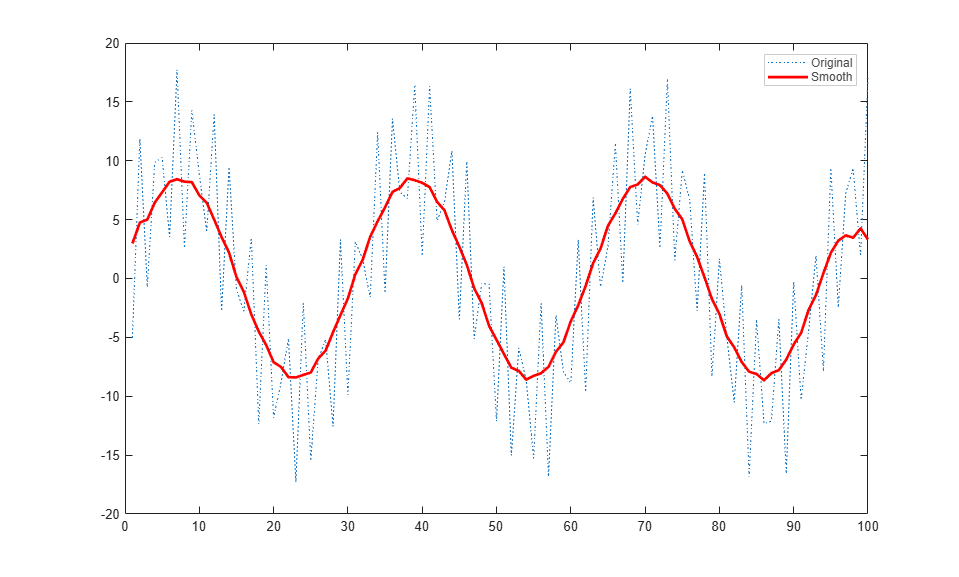# matlab.graphics.chartcontainer.mixin.Legend class

Package: matlab.graphics.chartcontainer.mixin

Add legend support to chart container subclass

## Description

`matlab.graphics.chartcontainer.mixin.Legend` is a class for adding legend support to charts that inherit from `matlab.graphics.chartcontainer.ChartContainer`. By inheriting from this class, you can display a legend in your chart. Your users can select options in the figure menu bar or the figure toolbar to show or hide that legend.

Use this syntax to enable legend support for your chart:

```classdef MyChart < matlab.graphics.chartcontainer.ChartContainer & ... matlab.graphics.chartcontainer.mixin.Legend ... end```

The `matlab.graphics.chartcontainer.mixin.Legend` class is a `handle` class.

### Class Attributes

 `Abstract` `true` `HandleCompatible` `true`

For information on class attributes, see Class Attributes.

## Properties

expand all

Legend visibility, specified as `'on'` or `'off'`, or as numeric or logical `1` (`true`) or `0` (`false`). A value of `'on'` is equivalent to `true`, and `'off'` is equivalent to `false`. Thus, you can use the value of this property as a logical value. The value is stored as an on/off logical value of type `matlab.lang.OnOffSwitchState`.

When this property is set to `'on'`, the legend is visible in the chart.

#### Attributes:

 `GetAccess` `public` `SetAccess` `public` `Dependent` `true` `NonCopyable` `true`

expand all

## Examples

collapse all

Define a `SmoothLegendPlot` class that plots a set of data using a dotted blue line with a smoothed version of the line.

To define the class, create a file named `SmoothLegendPlot.m` that contains the following code. The `setup` method for this class performs these tasks:

• Creates two `Line` objects with the appropriate `DisplayName` values to show in the legend

• Makes the legend visible by setting the `LegendVisible` property to `'on'`

• Gets the `Legend` object and customizes the text color, box edge color, and box line width

```classdef SmoothLegendPlot < matlab.graphics.chartcontainer.ChartContainer & ... matlab.graphics.chartcontainer.mixin.Legend properties XData (1,:) double = NaN YData (1,:) double = NaN SmoothColor (1,3) double {mustBeGreaterThanOrEqual(SmoothColor,0),... mustBeLessThanOrEqual(SmoothColor,1)} = [0.9290 0.6940 0.1250] SmoothWidth (1,1) double = 2 end properties(Access = private,Transient,NonCopyable) OriginalLine (1,1) matlab.graphics.chart.primitive.Line SmoothLine (1,1) matlab.graphics.chart.primitive.Line end methods(Access = protected) function setup(obj) ax = getAxes(obj); % Create line objects. Define line styles and legend names. obj.OriginalLine = plot(ax,NaN,NaN,'LineStyle',':',... 'DisplayName','Original'); hold(ax,'on') obj.SmoothLine = plot(ax,NaN,NaN,... 'DisplayName','Smooth'); % Make legend visible obj.LegendVisible = 'on'; % Get legend and set text color, edge color, and line width lgd = getLegend(obj); lgd.TextColor = [.3 .3 .3]; lgd.EdgeColor = [.8 .8 .8]; lgd.LineWidth = .7; hold(ax,'off') end function update(obj) % Update Line data obj.OriginalLine.XData = obj.XData; obj.OriginalLine.YData = obj.YData; obj.SmoothLine.XData = obj.XData; obj.SmoothLine.YData = createSmoothData(obj); % Adjust smooth line appearance obj.SmoothLine.LineWidth = obj.SmoothWidth; obj.SmoothLine.Color = obj.SmoothColor; end function sm = createSmoothData(obj) % Calculate smoothed data v = ones(1,10)*0.1; sm = conv(obj.YData,v,'same'); end end end ```

Next, create a pair of `x` and `y` vectors. Plot `x` and `y` by calling the `SmoothLegendPlot` constructor method, which is inherited from the `ChartContainer` class. Specify the `'XData'` and `'YData'` name-value pair arguments and return the chart object as `c`.

```x = 1:1:100; y = 10*sin(x./5)+8*sin(10.*x+0.5); c = SmoothLegendPlot('XData',x,'YData',y); ```Use `c` to change the color of the smooth line to red. Notice that the legend also shows the new line color.

```c.SmoothColor = [1 0 0]; ```## Version History

Introduced in R2019b